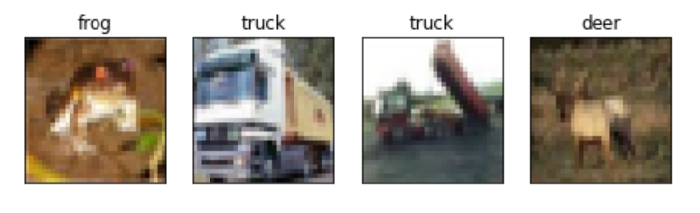# How to load a Computer Vision dataset in PyTorch?

There are many datasets available in Pytorch related to computer vision tasks. The torch.utils.data.Dataset provides different types of datasets. The torchvision.datasets is a subclass of torch.utils.data.Dataset and has many datasets related to images and videos. PyTorch also provides us a torch.utils.data.DataLoader which is used to load multiple samples from a dataset.

## Steps

We could use the following steps to load computer vision datasets −

• Import the required libraries. In all the following examples, the required Python libraries are torch, Matplotlib, and torchvision. Make sure you have already installed them.

import torch
import torchvision
from torchvision import datasets
from torchvision.transforms import ToTensor
import matplotlib.pyplot as plt
• We load CIFAR10 training and test datasets using datasets.CIFAR10() with the parameters train=True for training dataset and train=False for test dataset.

root="data",
train=True,
transform=ToTensor()
• Define a train dataloader (trainloader) and test dataloader (testloader). Specify the batch_size. Set Shuffle=True to get the shuffled images. Also access the class label names.

• Get some random images and labels from the training or test datasets.

dataiter = iter(trainloader)
images, labels = dataiter.next()
• Visualize the obtained images with the labels.

## Example 1

In the following Python program, we load CIFAR10 training and test datasets.

# Import the required libraries
import torch
import torchvision
from torchvision import datasets
from torchvision.transforms import ToTensor

# define batch size
batch_size = 4

training_data = datasets.CIFAR10(
root="data",
train=True,
transform=ToTensor()
)

test_data = datasets.CIFAR10(
root="data",
train=False,
transform=ToTensor()
)

# define train and test dataloader

# access names of the labels
label_names = training_data.classes

# display details about the dataset
print("label_names:", label_names)
print("class label name to index:", training_data.class_to_idx)
print("Shape of training data:", training_data.data.shape )
print("Shape of test data:", test_data.data.shape )

## Output

Files already downloaded and verified
label_names:
['airplane', 'automobile', 'bird', 'cat', 'deer', 'dog',
'frog', 'horse', 'ship', 'truck']
class label name to index:
{'airplane': 0, 'automobile': 1, 'bird': 2, 'cat': 3, 'deer':
4, 'dog': 5, 'frog': 6, 'horse': 7, 'ship': 8, 'truck': 9}
Shape of training data:
(50000, 32, 32, 3)
Shape of test data:
(10000, 32, 32, 3)

## Example 2

In this Python program, we load the CIFAR10 dataset. We also visualize some random images with their label names.

import torch
import torchvision
from torchvision import datasets
from torchvision.transforms import ToTensor
import matplotlib.pyplot as plt

batch_size = 4
training_data = datasets.CIFAR10(
root="data",
train=True,
transform=ToTensor()
)

test_data = datasets.CIFAR10(
root="data",
train=False,
transform=ToTensor()
)

label_names = training_data.classes

# get some random training images
images, labels = dataiter.next()

# display random images
# define figure
fig=plt.figure(figsize=(8, 5))
columns, rows = batch_size, 1

# visualize these random images
for i in range(1, columns*rows +1):
plt.imshow(images[i-1].numpy().transpose(1,2,0))
plt.xticks([])
plt.yticks([])
plt.title(label_names[labels[i-1]])
plt.show()

## Output

Files already downloaded and verified
Files already downloaded and verified